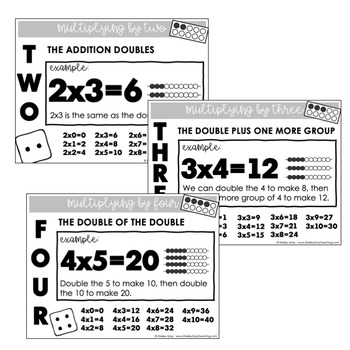# Multiplication Strategy Posters for Basic Multiplication Facts to 10x10Subject
Resource Type
File Type

PDF

(16 MB|14 pages)
Standards
Also included in:
1. Struggling to figure out how to properly teach multiplication and division, and continue to incorporate them into your classroom throughout the year?The Multiplication and Division Tool Kit will make this much easier for you! This special bundle includes loads of resources for teaching and reinforci
\$153.36
\$127.95
Save \$25.41
• Product Description
• StandardsNEW

Multiplication fluency is essential. When students are not fluent with multiplication facts, it creates difficulties with topics that rely on multiplication fact recall later.

Although automaticity is the goal, we want our students to really understand multiplication. True fluency occurs when students are not only quick with fact recall, but when they also are flexible thinkers who use appropriate strategies.

These posters will support multiplication strategies in your classroom. Use them throughout the year to teach and reinforce essential strategies.

If you already use The Multiplication Station, these posters will align with that resource and support your students' learning.

Solve multistep word problems posed with whole numbers and having whole-number answers using the four operations, including problems in which remainders must be interpreted. Represent these problems using equations with a letter standing for the unknown quantity. Assess the reasonableness of answers using mental computation and estimation strategies including rounding.
Multiply or divide to solve word problems involving multiplicative comparison, e.g., by using drawings and equations with a symbol for the unknown number to represent the problem, distinguishing multiplicative comparison from additive comparison.
Interpret a multiplication equation as a comparison, e.g., interpret 35 = 5 × 7 as a statement that 35 is 5 times as many as 7 and 7 times as many as 5. Represent verbal statements of multiplicative comparisons as multiplication equations.
Fluently multiply and divide within 100, using strategies such as the relationship between multiplication and division (e.g., knowing that 8 × 5 = 40, one knows 40 ÷ 5 = 8) or properties of operations. By the end of Grade 3, know from memory all products of two one-digit numbers.
Determine the unknown whole number in a multiplication or division equation relating three whole numbers. For example, determine the unknown number that makes the equation true in each of the equations 8 × ? = 48, 5 = __ ÷ 3, 6 × 6 = ?.
Total Pages
14 pages
N/A
Teaching Duration
N/A
Report this Resource to TpT
Reported resources will be reviewed by our team. Report this resource to let us know if this resource violates TpT’s content guidelines.